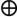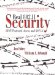# Example Message Modification

 For this example, we take the message above (ASCII 'N') and modify the message so it becomes an ASCII y and we still ensure that the ICV remains valid using the process described in Chapter 15. Stating the problem: Given P(x)= 01001110110, derive P'(x)=01111001XXX where XXX is a valid CRC. First, the delta between the current message, M(x), and the desired message, N(x), is computed by the exclusive OR of the two values. ` M(x) = 01001110 N(x) = 01111001 D(x) = 00110111 ` Now, the CRC is calculated for the delta value: ` ------------ 1101 |00110111000 1101 1100 1101 010 ` The remainder of the division is the CRC of the delta. Now the delta and its CRC are exclusive OR'd with P(x): ` P(x) 01001110110 D(x)00110111000 01111001110 CRC(D)010 P'(x) 01111001100 ` Now, P'(x) is shown to be a valid message; the remainder of the CRC calculation is 0. ` ------------ 1101 | 01111001100 1101 1000 1101 1011 1101 1101 1101 0 ` Because the remainder of the CRC calculation is 0, P'(x) has a valid CRC and the message has been successfully modified.Real 802.11 Security: Wi-Fi Protected Access and 802.11i
ISBN: 0321136209
EAN: 2147483647
Year: 2005
Pages: 151

Similar book on Amazon

flylib.com © 2008-2017.
If you may any questions please contact us: flylib@qtcs.net